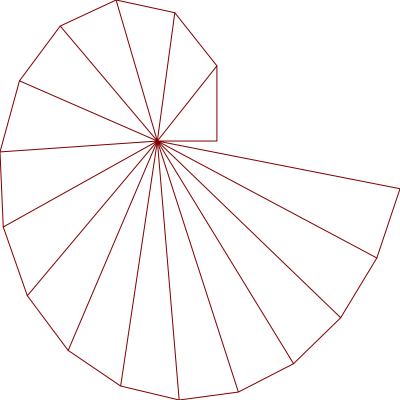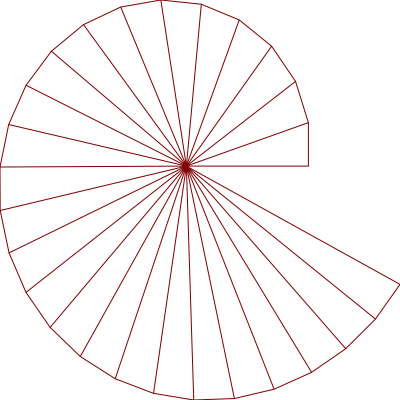# bsmall2 Learning Racket

## Plot a spiral

A Theodorus spiral with `plot` instead of `turtles`. The coding went quickly because it was easy to translate into Racket the math from Python code.The turtles version (fn:2)took a long time because I had to learn the math(fn:3) on my own.

### Python Code

The math part

``````    def next_vertex(x, y):
h = (x**2 + y**2)**0.5
return (x - y/h, y + x/h)
;  https://www.johndcook.com/blog/2019/08/04/spiral-of-theodorus/
``````

### Racket Code

``````#lang racket

(require plot)

(define (sqr n)
(* n n))

(define (next-vertex xy-vector)
(match-define (vector x y) xy-vector)
(define h (sqrt (+ (sqr x) (sqr y))))
(vector (- x (/ y h)) (+ y (/ x h))))

; plot lines for short-side and hypotenuse of triangle
(define (short-hypo-lines xy-vec)
(define new-xy
(next-vertex xy-vec))
(list (lines (list xy-vec new-xy))
(lines (list (vector 0 0) new-xy))))

;; Setting the N limit to 17 overlaps the first segment
(define N 16)
(define x-start 1)
(define y-start 0)

(define (spiral limit)
;; in place of `for` and `range` a recursive function with helper-auxiliary
(define (helper lim xy-vec lines)
(cond
((= 0 lim) lines)
(#t (helper (sub1 lim)
(next-vertex xy-vec)
(cons
(short-hypo-lines xy-vec)
lines)))))
;; starting plot at x-start, y-start
(helper limit (vector x-start y-start) '()))

; View in DrRacket or Emacs Geiser REPL
(parameterize
((plot-decorations? #f))
; in Python versionplt.axis('off')
(plot (list
; get started with first line from 0,0 to x-start, y-start
(lines (list (vector 0 0)(vector x-start y-start)))
;; plot the short-side and hypotenuse for the right triangles
;; that make form the spiral
(spiral N))))

; save plot to png file for on-line sharing
; ; from Python version  plt.savefig("theodorus.png")
(define spiral-bmp-1
(parameterize
((plot-decorations? #f))
(plot-bitmap (list
;; plot first line from 0,0 to x-start,y-start
(lines (list (vector 0 0)(vector x-start y-start)))
;; plot the short-side and hypotenuse for the right triangles
;; that form the spiral
(spiral N)))))

(send spiral-bmp-1 save-file "spiral-1-vector.png" 'png)

``````

Translating the math from Python gave me chance to use `match-define` and to get a feel for using vectors directly with `plot`. Earlier work with `plot` had me `map`ping `vector` over lists. My first script used lists and `list->vector`, then I saw that the code could be shortened by using vectors from the start ( `next-vertex` ) and adjusting all the code to work with `vector`. I still have to think about why `next-vertex` works.

Recursion in general and named let in particular provide a natural way to implement many algorithms, whether iterative, recursive, or partly iterative and partly recursive; the programmer is not burdened with two distinct mechanisms. — Kent Dybvig in The Scheme Programming Language Ch 3: Going Further

As an amateur programmer recursive functions with internal helpers seem to be the only structure (construct? pattern?) that I need. With recursive functions I am not “burdened with ... distinct mechanisms” and feel comfortable building the lists that are needed for various tasks. The recursive functions with `helper` functions may be the equivalent the of the Basic English (C.K. Ogden's) sentences at the start of English Through Pictures (I.A. Richards's): – I took the book off the table. – I put the book on the table. – I gave the book to her. – I went to the table. – I put the book in the box. – I took the book from the box.

``````(define N 26)
(define x-start 3)
(define y-start 0)
``````